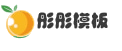通过织梦dedecms来调用论坛帖子的方法大集合

2021-07-29 0 2,450

今天我告诉大家一些在织梦DedeCMS里面调用discuz论坛帖子的一些方法，这些方法都是好不容易从网上搜来的，这次一次奉献给大家啦。

1、论坛最新主题调用代码如下：

·[field:subject function="cn_substr('@me',30)" /]([field:lastpost function="date('m-d H:M','@me')" /])</a>
<br/>
{/dede:loop}

这个连接地址你可以写相对路径，也可以写绝对路径。

如果是discuz动态论坛，链接地址如[field:tid /]

如果是discuz静态论坛，连接地址如[field:tid /]l

2、论坛指定版块帖子调用方法：

{ dede:loop table="cdb_threads" if="fid=1 or fid=2 and displayorder!=-1" sort="tid" row="6" }
<a href="/bbs/viewthread dot php?tid=[field:tid /]" target="_blank">
·[field:subject function="cn_substr(' @ me',50)" /] </a>
<br/>
{ /dede:loop }

其中的fid=1 or fid=2是论坛主题分类id，可以按照自己要求来修改，如果是一个分类就直接写fid=x

3、论坛调精华主题调用代码如下：

·[field:subject function="cn_substr('@me',30)" /]([field:lastpost function="date('m-d H:M','@me')" /])</a>
<br/>
{/dede:loop}

如果想调用某个板块的精华主题代码如下：

{dede:loop table="cdb_threads" if="fid=5 and displayorder!=-1" sort="tid" row="10"}
·[field:subject function="cn_substr('@me',30)" /]([field:lastpost function="date('m-d H:M','@me')" /])</a>
<br/>
{/dede:loop}

其中的fid=5是论坛主题分类id，可以按照自己要求来修改，

如果想调用论坛所有的板块的最新精华主题只要去掉fid=5 and 就可以了。

4、调用的帖子列表这可以按查看次数排序，代码如下：

·[field:subject function="cn_substr('@me',30)" /]([field:lastpost function="date('m-d H:M','@me')" /])</a>
<br/>
{/dede:loop}
其中sort="views" 就是控制按照查看次数排序的代码

5、论坛置顶版块帖子调用方法举例：

{ dede:loop table="cdb_threads" sort="dateline" if="fid='3' and typeid='51'" row="10" }
<a href="/a/bbs/viewthread dot php?tid=[field:tid /]" target="_blank">
·[field:subject function="cn_substr(' @ me',30)" /]</a> <br>
{ /dede:loop }

6、论坛版块名称调用方法：

{ dede:loop table="cdb_forums" sort="displayorder" row="16" col="4" }
<a href="/bbs/viewthread dot php?fid=[field:fid /]">·[field:name function="cn_substr(' @me',30)" /] </a>
{ /dede:loop }

注：这种方法这个还有点问题，它会将论坛分区、版块都调出来。望达人改进!

附：dedecms的LOOP 标记规则

功能说明：用于调用任意表的数据，一般用于调用论坛贴子之类的操作

适用范围：所有模板

基本语法:

{ dede:loop table=' sort='' row='' if='' }

 table 表示查询的数据表

 sort 用于排序的字段 也可以加"views"(查看次数排列)

 row 返回结果的条数

 if 查询条件

7、调用论坛帖子中的图片方法，网上搜到的，我自己还没有做测试。

ON`cdb_threads`.`tid`=`cdb_attachments`.`tid` WHERE `cdb_attachments`.`readperm`='0' AND`displayorder`>='0' AND `filetype`='image/pjpeg' GROUP BY tid LIMIT 0,1 "}
<img src="/bbs/attachments/[field:attachment/]" ALT="[field:subject/]" width="100" height="80" border="0" />
</A>
{/dede:sql}• 模板团承诺演示效果图均和网站95%一样，并且我们的模板和数据都没有留后门，请放心使用。查看详情
• 因为模板具有可复制性和可传播性，模板一旦发货，非质量问题，不支持退换；所以务必请您一定看清楚后再购买。查看详情

2周前 2,744
2周前 4,819
2周前 3,574
6个月前 2,842

• 0 +

访问总数

• 0 +

会员总数

• 0 +

资源总数

• 0 +

今日发布

• 0 +

本周发布

• 0 +

运行天数